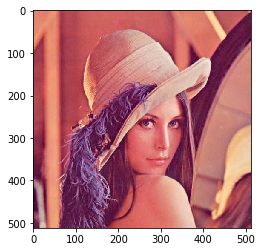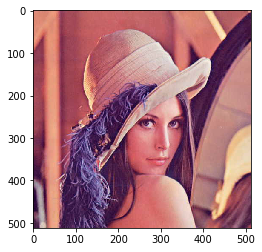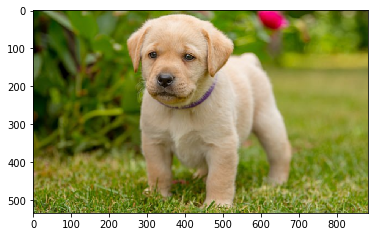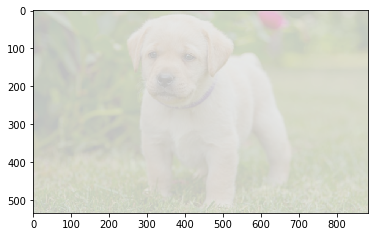GFG App
Open AppBrowser
Continue

# Mahotas – Image Stretch RGB

In this article we will see how we can do stretching for RGB image in mahotas. Contrast stretching (often called normalization) is a simple image enhancement technique that attempts to improve the contrast in an image by `stretching’ the range of intensity values it contains to span a desired range of values, e.g. the full range of pixel values that the image type concerned allows. RGB stretching is variation of stretch() function that works per-channel on an RGB image

In this tutorial we will use “lena” image, below is the command to load it.

`mahotas.demos.load('lena')`

Below is the lena imageIn order to do this we will use mahotas.regmin method
Syntax : mahotas.stretch_rgb(img)
Argument : It takes image object as argument
Return : It returns image object

Below is the implementation

## Python3

 `# importing required libraries` `import` `mahotas` `import` `mahotas.demos` `from` `pylab ``import` `gray, imshow, show` `import` `numpy as np` `import` `matplotlib.pyplot as plt` `  `  `# loading image` `img ``=` `mahotas.demos.load(``'lena'``)` `  `  `print``(``"Image"``)` `  `  `# showing image` `imshow(img)` `show()`   `# stretching rgb image` `new_img ``=` `mahotas.stretch_rgb(img)` ` `    `# Stretched image` `print``(``"Stretched Image"``)` `imshow(new_img)` `show()`

Output :

`Image``Stretched Image`Another example

## Python3

 `# importing required libraries` `import` `mahotas` `import` `numpy as np` `from` `pylab ``import` `gray, imshow, show` `import` `os` `import` `matplotlib.pyplot as plt` ` `  `# loading image` `img ``=` `mahotas.imread(``'dog_image.png'``)`   `  `  `print``(``"Image"``)` `  `  `# showing image` `imshow(img)` `show()`   `# stretching image` `new_img ``=` `mahotas.stretch_rgb(img)` ` `    `# Stretched image` `print``(``"Stretched Image"``)` `imshow(new_img)` `show()`

Output :

`Image``Stretched Image`My Personal Notes arrow_drop_up